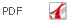J. Iran. Chem. Soc., Vol. 8, No. 3 September 2011, pp.872~878.

Current location: JICS Archive > Vol. 8 > No. 3 > Articles : 28

# The Exact Determination of the Critical Points for 2-D Square Lattice Ising Model with the Nearest and Next Nearest Neighbors Interactions

## S. Ranjbar

### Chemistry Department, Science College,Razi University, Kermanshah, Iran

The combinatorial factor method (CFM) was used to find the critical points of the 2-D square lattice Ising model with the nearest neighbor (with the coupling constant Jnn) and the next nearest neighbor (with the coupling constant Jnnn) interactions. In the absence of the magnetic field, for the various values of coupling ratio, α = Jnnn/Jnn (0 ≤ α < 1), the exact values of  jc (= Jnn/kTc) were obtained. Finally, based on such values, an analytical expression for the jc as a function of α has been proposed

Keywords: Ising model, Combinatorial factor, Phase transition, Nearest- and next nearest-neighbors